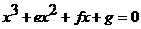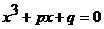Every cubic equation in the form

(1)can be expressed in the form

(2)by dividing every term by a.

Every equation in the form of (2) can be expressed in the form

(3)by substitutingfor x into equation (2)

The three solutions to equation (3) are